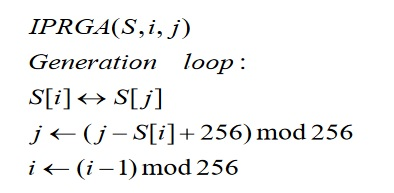# CS 435/890BN Programming Assignment

An RC4 state is a 256 bytes states with two 8-bit index pointers i and j denoted by (S, i, j). The initial RC4 state is generated by KSA denoted by (S0, i = 0, j = 0).

An important feature of RC4 is that the RC4 state is reversible. That is, if (S*, i*, j*) = PRGAn (S, i, j), it has (S, i, j) = IPRGAn (S*, i*, j*) where PRGAn denotes applying n rounds PRGA (same for IPRGAn) and IPRGA is the reverse algorithm of PRGA. This feature means that any former RC4 state can be recovered from a later RC4 state by applying certain rounds IPRGA.Write code to implement both the PRGA algorithm and the above IPRGA algorithm anddesign and implement a way to confirm that any RC4 state can move forward by PRGA andbackward by IPRGA. Please note, Part One is not asking you to implement the encryption and decryption of using RC4.

Note:

1. Both source code and related documentation for part one are required. Please compress your all files for part 1 into one file with name PA1-YourName-SID and submit through UR Courses.
3. You can use any common programing language and tool to implement it.

Part Two (Weight: 75%)

An RC4 state based secure unicast protocol is described in the following: Suppose A (sender) and B (receiver) have the same secure key (128 bits) to initialize RC4 state (S, i, j)A for A and (S, i, j)B for B and initially (S, i, j)A = (S, i, j)B = (S, i, j)o. Initially A and B also set their sequence counters to zero. Each data packet has 272 bytes (4 bytes for sequence counter value, 252 bytes for data segment and 16 bytes for hash value):

Data Segment (252 bytes)

HV (16 bytes)

1

For Sender:

1. The sender divides the input plaintext message into contiguous 252-byte data segments and assigns SC to each of them. The sequence counter (SC) value is increased by 1 in increased order (initially SCA = 0). If there are not enough data in the data segment of the last data packet, pad a 1 followed by as many 0 as necessary.
2. The sender calculates the hash value for that data packet by inputting SC and the unencrypted data segment, and then places the 128-bit hash value into the data packet.
3. The sender produces the encrypted data packets by only encrypting data segment and hash value (do not encrypt SC value). The sender updates its SCA and (S, i, j)A after the encryption.

Initially (S, i, j)B = (S, i, j)0 and SCB = 0. When receiving a new packet, B compares its own SC value (SCB) with the SC value of the packet. If the difference of the SC value of the packet and its own SC value (SCpacket - SCB) is 0, then (S, i, j)B is used as the RC4 state to decrypt the data segment and hash value of that incoming packet and then increase the sequence counter by 1. Otherwise, calculate the right RC4 state from current (S, i, j)B by applying certain rounds of PRGA or IPRGA, and then use the right RC4 state to decrypt the data segment and hash value of that incoming packet and set the sequence counter value of receiver by the SC value of the packet plus 1. B also needs to calculate the hash value according to the decrypted data (SC and data segment) and then compare it with the one directly gets from decrypted packet (comparison is required, but assume it always match).

Write a program with two interfaces (one for sender and another for receiver) to implement the above secure unicast protocol.

Test your program by a 1000 bytes message (4 packets). Suppose:

Case 1: the sequence of the packets received is 0, 1, 2 and 3

Case 2*: the sequence of the packet received is 1, 0, 3 and 2

Case 3*: the sequence of the packet received is 3, 2, 1 and 0

* in case 2 and case 3, both PRGA and IPRGA should be used to achieve the requirement.

Note:

1. Both source code and related documentation for part two are required. Please compress your all files for part 2 into one file with name PA2-YourName-SID and submit through UR Courses.